﻿ Application of Factor Analysis on Academic Performance of PupilsPublications are Open
Access in this journal
Article Versions
Export Article
• Normal Style
• MLA Style
• APA Style
• Chicago Style
Research Article
Open Access Peer-reviewed

### Application of Factor Analysis on Academic Performance of Pupils

Okeke Evelyn Nkiruka , Okeke Joseph Uchenna, Yawe Ayuba, Anule Josaphat, Bello Abdulrasheed
American Journal of Applied Mathematics and Statistics. 2017, 5(5), 164-168. DOI: 10.12691/ajams-5-5-2
Published online: December 07, 2017

### Abstract

Over the years, efforts have been made by researchers in studying the effects of certain factors on academic performance of students, though with little concentration on the primary section of education. This research therefore worked on the academic performance of pupils in primary school. Variation in the analyzed performance and the factor(s) causing the variations were studied using factor analysis. The data used is a secondary data, collected from Federal University Wukari Staff School and it’s on the terminal examination scores of the pupils in seven selected subjects over one selected academic session (2015/2016). From both the un-rotated and rotated factor analysis results, we observed a fair relationship between the mathematical and less mathematical subjects, though they present the major variation in the pupils’ performance to be in the less mathematical subjects like English Language, Verbal Aptitude, Social Studies, Creative Art, and Religious Studies. Also, the analysis presented three factors (gender, age, and environment) to be the cause of the variation between the pupils performance in primary school.

### 1. Introduction

Factor analysis is a multivariate method that studies the variability among observed correlated variables in terms of a potentially lower number of unobserved factors that are supposed to contain essential information in a larger set of observed variables. The factors are constructed in a way that reduces the overall complexity of the data by taking advantage of inherent interdependencies. As a result, a few numbers of factors will usually account for approximately the same amount of information as do the much larger set of original observations.

The broad purpose of factor analysis is to summarize data so that relationships and patterns can be easily interpreted and understood. It is normally used to regroup variables into a limited set of clusters based on shared variance. Hence it helps to isolate constructs and concepts Yong and Pearce 1. In factor analysis observed variables are being represented as the linear combination of the unobserved factors.

Richard and Dean 2 declared that the essential purpose of factor analysis is to describe, if possible, the covariance relationship among many variables in terms of a few underlying but unobservable random quantities called factors.

There are two main types of factor analysis; the exploratory factor analysis (EFA) and confirmatory factor analysis (CFA) 3. According to him exploratory factor analysis (EFA) is data driven, such that the collected data determines the resulting factors while confirmatory factor analysis (CFA) is used to test factors that have been developed a priori.

Ballester et al 4 compared the performance of principal component analysis and factor analysis and stated that principal component analysis is recommended for dimensional reduction, whereas, factor analysis is adopted for the study of structures in data. Principal component analysis is a linear combination of variables while factor analysis is a measurement mode of a latent variable Karen 3.

This research work is aimed at evaluating pupils’ performance with respect to the subjects that determine their performance using factor model.

### 2. Factor Analysis

2.1. Type of Factor Analysis

We have two types of factor analysis and this include

• Exploratory Factor Analysis

• Confirmatory Factor Analysis

Exploratory factor analysis (EFA) is data driven; here the data collected determines the resulting factors. This analysis tries to uncover complex patterns by exploring the dataset and testing prediction 5.

Confirmatory factor analysis (CFA) is used to test factors that have been developed a priori 3. It’s seen as a process for testing what you already think you know. CFA is an integral part of structural equation modeling (SEM) and path analysis. It attempts to confirm hypothesis and use path analysis diagrams to represent variables and factors. CFA is also a useful tool in checking the reliability of a measurement tool with a new population of subject, or to further refine an instrument which is already in use

2.2. Condition for the Use of Factor Analysis

• Factor analysis is usually performed on ordinal or continuous variables, although it can also be performed on categorical and dichotomous variable.

• Data that will be used to perform factor analysis has to be univariate and multivariate normal 5 and free from univariate and multivariate outliers 6

• A determining factor is based on the assumption that there is a linear relationship between the factors and the variable when computing the correlations 7. For something to be called a factor it must at least has three variables, although this depends on the design of the study 8. A factor with 2 variables is only considered reliable when the variables are highly correlated with each other (r > 0.70) and 2 or fewer variables should be interpreted with caution.

• The sample size that should be considered for factor analysis should be at least 30 and the factors should have at least 5 to 10 observations each 9.

• It is also recommended that a heterogeneous sample is used rather than a homogeneous sample as homogeneous samples lower the variance and factor loadings 10. Also the correlation, r, existing between the variables must be 0.30 or greater since anything lower would suggest a really weak relationship between the variables 8.

• Generally speaking, cases with missing values are deleted to prevent overestimation 8.

• There should be absence of multicollinearity and singularity with the dataset. Variables that have issue with singularity and multicollinearity should be removed from the dataset.

2.3. Factor Analysis Model

Factor analysis is a statistical method of investigating whether a number of variables of interest, say,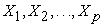are linearly related to a number of unobservable variable called factors, say,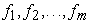where m is relatively less than p, that is,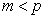.

Given observations on p-variables,with mean vectorand covariance matrix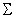. Noting that the factorsare independent of one another and of the error termsfor, and are such that,and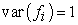, and holding to the fact that each of the observed variable is a linear function of these factors and error term, we have the factor analysis model for a variable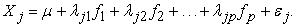(1)

Noting that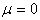when the observations are standardized we have (1) to be written as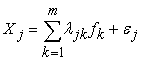(2)

whereis the factor loading, such that,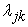is loading of the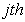variable on thefactor,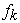are the underlying variables, the specific or unique factors is denoted by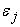,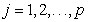and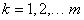.

The factor loading give us an idea about how much the variable has contributed to the factor; the larger the factor loading the more the variable has contributed to the factor Harman 11. Factor loading are very similar to weights in multiple regression analysis, and they represent the strength of the correlation between the variable and the factor 10.

Equation (1) can be expressed in matrix form as;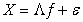(3)

whereThe variance of (1) we give(4)

Since it has been stated that the factorhas unit variance, hence (3) can be written as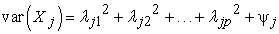(5)where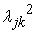is the kth cummunality, and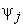the jth specific variance.

The covariance of X denoted bycan be expressed as:Since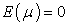Recalling thatwe have that(6)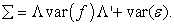Since the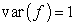, we have that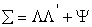(7)

Where2.4. Estimation of Factor Score

Factor score are the score of each of the variables on each factor. To compute the factor score for a given variable of a given factor, one takes the standardized score on each variable multiplied by the corresponding factor loading of the variable for the given factor, and sums these products. A number of methods are being used to estimate the factor scores, for this research work, the method of ordinary least squares will be employed. Using factor model from (3) above, we have that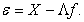(8)

Squaring both sides of (8) and taking summation gives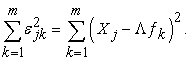Minimizing the above equation with respect to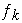and setting the derivative to zero givessince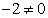, then the expression becomes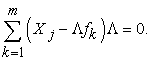This then givesDividing the equation above by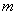and setting it in terms ofwe haveMultiplying the equation above by inverse of the coefficient ofwe have that;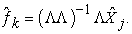(9)

Equation (9) can therefore be referred to as factor scores.

The number of factors to be retained in the work will be determined using scree plot and principal component analysis method.

2.5. Factor Rotation Methods

Factors are rotated for better interpretation of results since unrotated factors are ambiguous. The goal of rotation is to attain an optimal simple structure which attempts to have each variable load on as few factors as possible, but maximizes the number of high loading on each variable 8. Ultimately, the simple structure attempts to have each factor define a distinct cluster of interrelated variables so that interpretation is easier. In this research work we considered four different rotational methods which include equamax, verimax, quartimax, and orthomax (at gamma 0.00 and 1.00 values). Among these are orthogonal and oblique rotations. Orthogonal rotation is when the factors are rotated 90° from each other, and it is assumed that the factors are uncorrelated 12, 13. Oblique rotation is when the factors are not rotated 90° from each other, and the factors are considered to be correlated. Oblique rotation is more complex than orthogonal, since it can involve one of two coordinate systems: a system of primary axes or a system of reference axes 13.

Quartimax rotation - is an orthogonal technique that involves the minimization of the number of factors needed to explain each variable 7.

Verimax rotation - is an orthogonal technique that minimizes the number of variables that have high loadings on each factor and works to make small loadings even smaller.

Equamax rotation –is orthogonal rotation invented with intention of sharpening some properties of varimax. It is self-adjusted for the number of the being rotated factors. It tends to distribute variables (highly loaded) more uniformly between factors than verimax does and thus further is less prone to giving “general” factors.

Orthomax – is a procedure for simple structure rotation of a matrix, that is, a two-way array. A loading matrix has perfect simple structure if each row has at most one nonzero element. It is shown that there is an orthogonal rotation of an initial loading matrix that has perfect simple structure, and then orthomax rotation with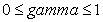of the initial loading matrix will produce the perfect simple structure 14. Gamma specifies the member of the family to use. Varying Gamma changes maximization of the variances of the loading from column (Varimax) to rows (Quartimax).

### 3. Academic Performance of Pupils

Factor analysis of pupils’ performance is a form of exploratory multivariate analysis that is used to either reduce the number of variables in a model or to detect relationship among variables affecting academic performance of pupils. Many studies have been conducted in the area of academic performance and those studies identified some number of factors that affect academic performance of pupils at primary school, students at college and even at university level. According to Epatein et al., 15, Wing et al., 16, Uphoff and Gilmore 17, Anene 18, academic performance has been known to be affected by a number of factors such as learning style, age, gender, teacher factor, location, economic status of parents, and many others.

Coley 19 found that females scored higher than males in reading and writing across different ethnic and age group. Also Alton-Lee and Praat 20 and Mullis et al 21 noted that educational statistics have indicated that females outperformed males at all levels of the school system, attaining school and post school qualification and attending university at higher numbers.

De Meis and Steams 22 and Diwtz and Wilson 23 found no significance relationship between age and academic performance. In contrast, La Paro and Pianta 24 and Crosser 25 presented evidence that older children fare better academically than their younger age appropriate peers.

According to Kibra and Kikomoti 26, the language spoken at the homes of the people of high class is often the medium of instruction in school; hence children from this class have advantage over children who speak their mother tongue at homes.

### 4. Methodology

For proper and effective analysis of this research work, the Federal University Wukari Staff School is selected as a case study. The data used is a secondary data collected from the school; it is on the outcome of grades scored by pupils of the school through the terminal examination conducted by the school. It is collected from primary one to primary five by a random selection of terms from the 2015/2016 academic session. The sample of size 9 was randomly selected from each class making a total of 45 observations. The information on the factors and observed variables mentioned above were collected from a random selection across the classes of the school.

In this study, the pupils’ scores per 100 percent in selected subjects which are English Language, Mathematics, Social Studies, Religious Studies, Verbal Reasoning, Quantitative Reasoning and Creative Arts are the observed correlated variables and their age, gender and location are the factors.

### 5. Result and Discussion

From the correlation matrix of the entire data we observed the variables are correlated and that the correlations among the variables are relatively fair, ranging from 0.358 to 0.762 which indicates no singularity problem in the data.

From the Bartlett’s test for the homogeneity of variance we observed the Kaiser-Meyer-Olkin value of 0.851 which falls within the range of superb (0.8-0.9) according to Hutcheson and Sofroniou 27, which implies that the variances across the variable are not equal. In this regard, this calls for the use of factor analysis.

From the Table of communalities which measures the percentage of variance explained by all the components before and after extraction we observed that 75.7% of the variance associated with English language was extracted; 75.8% of the variance associated with Verbal reasoning extracted; 81.0% of the variance associated with Social studies extracted and so on. The extracted communalities is the percentage of variance in a given variable explained by the factors that are extracted, which are normally fewer in number than the original variable.

From the scree plot and Table of Total variance explained, we observed that three out of seven factors accounted for 85.06% of the total variance in the data, hence, it can be seen that three factors are responsible for the variation in the original data used. A factor’s eigen value may be computed as the sum of its square loading for the entire variable.

From the unrotated factor loadings we observed that Social Studies, verbal attitude, English language and creative art have high loading in the three selected factors. This goes a long way to prove that location and economic class of a child have influence in his academic performance. It also tells us that art subjects are main subjects that mainly determine the pupils’’ performance at the end of examination.

When we considered equamax, verimax and orthomax (at gamma =1.00) rotations we observed that creative act, religious studies and quantitative reasoning are highlighted by the three selected factors, which goes a long way to indicate that age has a lot to do with the pupils’ performance.

From quartimax rotation and orthomax rotation (at gamma = 0.00) we observed that verbal attitude, English language, social studies, creative art, and religious studies are subjects that determine the position of pupil at the end of examinations. Since at primary school male pupils are good in creating thing like constructing of kits, toy car, catapults and female pupils are more social we can deduced from this that gender of a pupil has influence in his academic performance.

These discoveries are in line with what we have in the literature under academic performance of pupils.

### References

  Yong, A. G. and Pearce, P. (2013). A beginner’s guide to factor analysis: Focusing on exploratory factor analysis, Tutorials in quantitative methods for Psychology, 9(2): 79-94. In article View Article  Richard, A. and Dean, W. (1992). Applied multivariate analysis, Pretence Hall Englewood Cliffs, New Jersey 07632 In article  Karen, G. (2017). Confirmatory Factor analysis, Webiner, http://www.theanalysisfactor.com/february-2017-cfa/. In article View Article  Ballester, M.A., Linguraru, M.G., Aguirre, M.R., and Ayache, N. (2004). On the adequacy variability, Epidaure Research Project 2004 route deslucioles BP3, Sophia Antipolis, 06902, France. In article  Child, D. (2006). The essentials of factor analysis in behavioral and life science, New York, NY: Plenum Press. In article  Field, A. (2009). Discovering statistics using SPSS: Introductory statistical method (3rd ed.). Thousand Oaks, CA: Sage Publication In article  Gorsuch, R.L. (1983). Factor analysis (2nd ed.). Hillside NJ Lawrence Eribaum Associate. In article PubMed  Tabachnick, B. G., and Fidell, L. S.(2007). Using Multivariate statistics (5th ed.). Boston, MA: Allyn and Bacon. In article PubMed  Comrey, L.A., and Lee, H.B. (1992). A first course in factor analysis (2nd ed.). Hillside NJ Lawrence Eribaum Associate. In article PubMed  Kline, P. (1994). An easy guide to factor analysis, Ney York NY: Routledge. In article View Article  Harman, H.H. (1976). Modern factor analysis (3rd ed. revised), Chicago, IL: University of Chicago Press. In article PubMed  Decoster, J. (1998). Overview of factor analysis, Retrieved march 22, 2012 from http://www.stat-help.com/notes. html. In article View Article  Rummed, R. J. (1970). Applied factor analysis, Evanston, II Northwestern University Press. In article View Article  Bernaards, C. A. and Jennrich R. I. (2003). Orthomax rotation and perfect simple structure, Psychometrika, 68(4): 585-588. In article View Article  Epatein, D., Elwood, J., Hey, V. and Maw, J. (Eds). (1998). Failing Boys? Issue in gender and achievement, Buckingham: Open University Press. In article View Article  Wing, L., Leekam, S., Libby, S., Gould, J., and Larcombe, M. (2002). Diagonostic interview for social and communication disorders: Background, inter-rater reliability and clinical use, Journal of child Psychology and Psychiatry, 43:307-325. In article View Article  PubMed  Uphoff, J. K. and Gilmore, J. (1985). Pupils’ age at school entrance, how many are ready for success? Educational Leadership, 43: 86-90. In article View Article  Anene, G. U. (2005). Home environment and the academic performance of a chikd, Journal of Home Economics Research, 6(1): 99-100. In article  Coley, R. (2001). Difference in the gender gap: Comparisons across racial/ethnic group in education and work, Princeton Education Center, www.ets.org/research/pic. In article View Article  Alton-Lee, A. and Praat, A. (2001). Explaining and addressing gender differences in the New Zealand compulsory school sector, Wellinton: Ministry of Education. In article  Mullis, I. V. S., Martin, M. O., Gonzalez, E. J. and Kennedy (2003). PIRLS 2001 international report: IEA’S study on reading achievement in primary schools. Chestnut Hill, MA: Boston College. In article  DeMeis, J. I. and Stearns, E. S. (1992). Relationship of school entrance age to academic achievement, Journal of Educational Research, 86(1):20-27. In article View Article  Diwtz, C. and Wilson, B. J. (1985). Beginning school age and academic achievement, Psychology in the Schools, 22(1):93-94. In article View Article  La Paro, K. M. and Pianta, R. C. (2010). Predicting children’s competence in the early school years: A mete-analysis review, Review of Educational Research, 70(4):443-484. In article View Article  Crosser, S. L. (1991). Summer birth date of children, Kindergarten entrance age and academic achievement, Journal of Educational Research, 84(3):140-146. In article View Article  Kibera, L.W. and Kikomoti, A. (2007). Fundamental of Sociology and education with reference in Africa, Nairobi: University of Nairobi Press. In article  Hutcheson, G. D. and Sofronious, N. (1999). The multivariate social scientist: An introduction to generalized linear models, Sage Publications. In article View Article  PubMed

Published with license by Science and Education Publishing, Copyright © 2017 Okeke Evelyn Nkiruka, Okeke Joseph Uchenna, Yawe Ayuba, Anule Josaphat and Bello AbdulrasheedThis work is licensed under a Creative Commons Attribution 4.0 International License. To view a copy of this license, visit http://creativecommons.org/licenses/by/4.0/